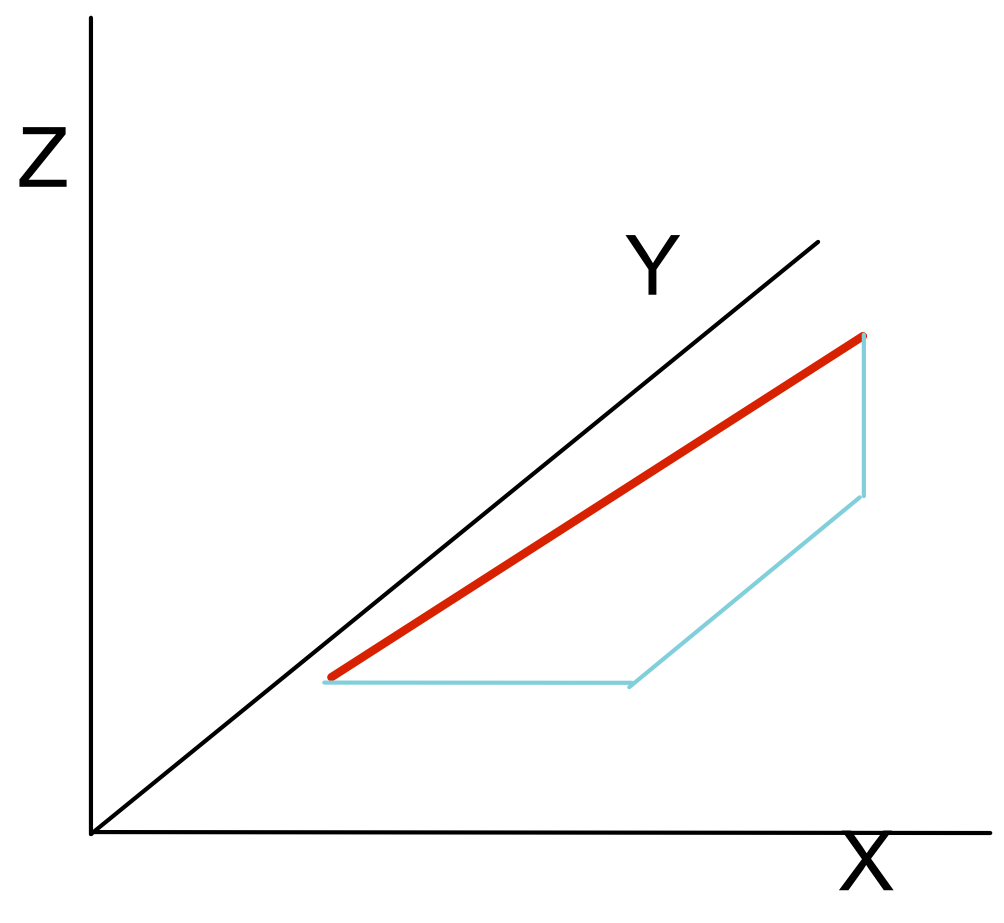3D Vectors

## 3D Vectors

3D Vectors behave in the same way as 2D Vectors, except that there is a third dimension to take into account.

For a column vertex, the z-direction is added as a third row eg vec(AB) = ((3),(5),(7)), where the 7 is the movement in the z-direction.

The magnitude of the vector is given by Pythagoras Theorem applied in three dimension.## Example 1

Point A is a point on a three dimension graph at position (1, 2 ,3). Point B is a point on the same graph at position (4, 5, 6). Show vec(AB) as a column vector.

The x value is 4 - 1 = 3

Similarly, y is 5 - 2 = 3 and z is 6 - 3

Answer: ((3),(3),(3))

## Example 2

Vector vec(AB) = ((3),(4),(5)). What is the magnitude of vec(AB)? Give your answer to 2 decimal places.

Use Pythagoras Theorem in 3D.

 Pythagoras a^2 = b^2 + c^2 + d^2 substitute a^2 = 3^2 + 3^2 + 3^2 a = sqrt(27) a = 5.196 2 dp a = 5.20